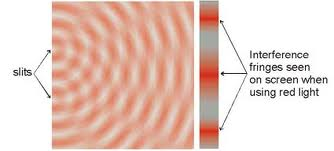Tuesday, 17 September 2013

Approximate Wavelength Formula, λ = ax/D

Approximate Formula for Wavelength (λ = ax/D) from Interference Pattern:
• Where, λ = wavelength
a = distance between the 2 coherent wave sources

x = distance between 2 adjacent antinodal lines
(radiating ripples or interference fringes or points of loudness)

D = perpendicular distance between the parallel lines
where a and x are  measured
• Empirical evidence from experiment shows:
• that x is directly proportional to λ
• that x is inversely proportional to a
• therefore, mathematically, x = kλ/a, where k is found to be = D
• Thus, x = Dλ/a
• And, changing the subject of formula, we have: λ = ax/D
• Application 1: The "Approximate Wavelength Formula, λ = ax/D" can be used to find the wavelength of waves from their interference patterns as a, x and D can be determined from the interference pattern.
• Application 2: From the same formula λ = ax/D or x = Dλ/a (same formula with different subjects)
• The interference pattern of red, green or blue light can all be explained by the "Approximate Wavelength Formula, x = Dλ/ax red > xblue• The distance between adjacent fringes x of red light is bigger than that of green or blue light because the wavelength of red light (λ red) is longer as compared to that of green light (λgreen) or blue light.
• Since  λ red > λgreen > λblue, from  x = Dλ/a, therefore, x red > xgreen > xblue.
--------------------------------------------------------------------------------

Need Help In Physics, Chemistry and Maths in or around Petaling Jaya?

Good News: Vacant tuition slots are now up for grab!

(May 30th, 2016): Summer May/June 2016 IGCSE O-level and CIE AS/A2 exams are ending. As such, some tuition slots are available for grabs on '1st-come-1st-serve". The tuition slots are for:

* IGCSE: Math 0580
Math 0606
Physics 0625
Chemistry 0620

(Edexcel Maths and Physics; SPM Maths, Physics and Chemistry - hourly rates: min. RM80/hr 1-to-1 or RM50/hr/student for group of 2 to 4)

* CIE AS/A2: Chemistry 9701 (min RM110/hr 1-to-1)

WhatsApp me Douglas at: 018-3722 482 or,
email: tutortan1@gmail.com

Thank you.
-----------------------------------------------------------